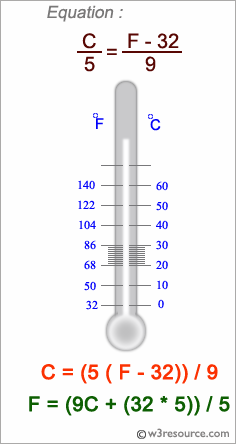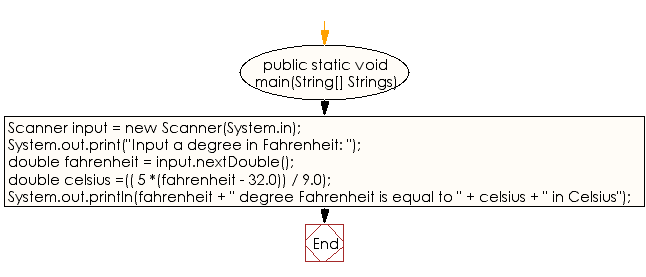﻿ Java exercises: Fahrenheit to Celsius degree - w3resource# Java Data Type Exercises: Fahrenheit to Celsius degree

## Java Data Type: Exercise-1 with Solution

Write a Java program to convert temperature from Fahrenheit to Celsius degree.

The Fahrenheit scale is a temperature scale based on one proposed in 1724 by physicist Daniel Gabriel Fahrenheit. It uses the degree Fahrenheit (symbol: °F) as the unit.

The Celsius scale, previously known as the centigrade scale, is a temperature scale used by the International System of Units (SI). As an SI derived unit, it is used by all countries in the world, except the U.S.

Test Data
Input a degree in Fahrenheit: 212Sample Solution:

Java Code:

``````import java.util.Scanner;
public class Exercise1 {

public static void main(String[] Strings) {

Scanner input = new Scanner(System.in);

System.out.print("Input a degree in Fahrenheit: ");
double fahrenheit = input.nextDouble();

double  celsius =(( 5 *(fahrenheit - 32.0)) / 9.0);
System.out.println(fahrenheit + " degree Fahrenheit is equal to " + celsius + " in Celsius");
}
}
```
```

Sample Output:

```Input a degree in Fahrenheit: 212
212.0 degree Fahrenheit is equal to 100.0 in Celsius
```

Flowchart:Java Code Editor:

Improve this sample solution and post your code through Disqus

What is the difficulty level of this exercise?

﻿

## Java: Tips of the Day

Checks if a string is upper case:

```public static boolean isUpperCase(String input) {
return Objects.equals(input, input.toUpperCase());
}
```

Ref: https://bit.ly/39Hpo84